02 Aug 2016
August 2, 2016

## Calculating Berthing Energy 2.c)

0 Comment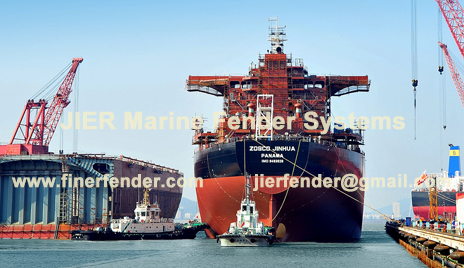##### Calculating Berthing Energy 2.c)

C) Eccentricity- Ce

Usually the ship is not parallel to the pier face during berthing. As a result, not all of the Kinetic Energy will be transmitted to the fenders. At impact, the ship will start to rotate around the contact point thus dissipating part of its energy.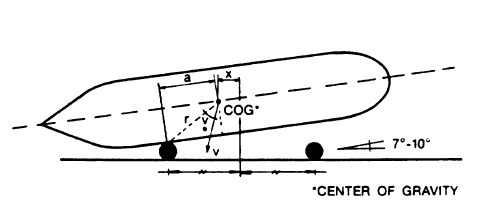The following graph illustrates the relationship between the eccentricity coefficient and the distance “a” (as shown above).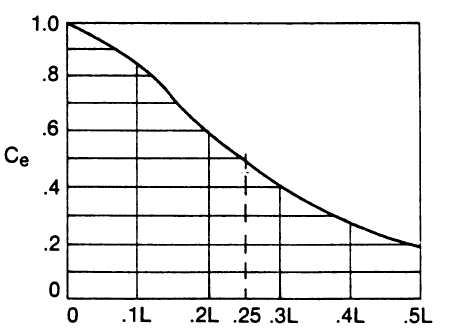Alternatively, it is represented by the formula: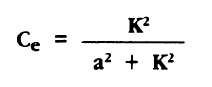Where:

• K = radius of longitudinal gyration of the ship
• a = distance between the ship’s centre of gravity and the point of contact on the ship’s side projected onto the longitudinal axis (in terms of L – the ship’s length)

The value of K is related to the block coefficient of the ship and its length. It can be approximated by the following expression: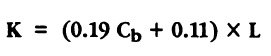and the block coefficient Cb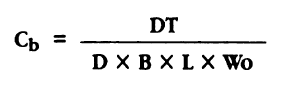Where:

• DT = Displacement of the ship (tonnes)
• D = Draft (m)
• B = Width (m)
• L = Length (m)
• Wo = Water Density (tonnes/M3)

Typical Seawater Wo = 1.025 tonnes/M3 (64 lb/ft3)

Typical Freshwater Wo = 1.00 tonnes/M3 (62.3 lb/ft3)

For larger Bulk Ships and Tankers

K = 0.2L – 0.25L

For Passenger Ships and Ferries

K = 0.17L – 0.2L

For 1/4 point Berthing a = 0.25L

The formula is based on the generally accepted assumptions that at the moment of maximum fender deflection:

1. Rotation only occurs at the contact point
2. Ship’s hull does not slide along the fender
3. Forces such as wind, currents tugs are negligible compared to the fender reaction.

The approach angle is usually taken as 7°with a maximum of 10 °. If the ship is berthing properly under control at the moment of contact with the fender then the direction of travel will be at right angles to the berthing face.

Examples:

In the case of a two dolphin mooring where the dolphins are 1/3 L distance apart, the minimum Ce is reached when the center of gravity of the large ship falls halfway between the two dolphins on contact with the fenders. This is when a = 1/6 L

Therefore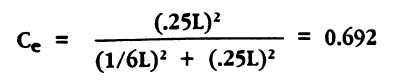The maximum in this case, would occur when the ship’s center of gravity falls in line with the point of contact with the fender or a = 0 then Ce = 1.

In the case of a continuous fender system and a large oil tanker a = 0.3L

Therefore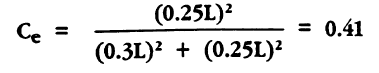Generally Ce ranges between 0.4 and 0.8

[top]